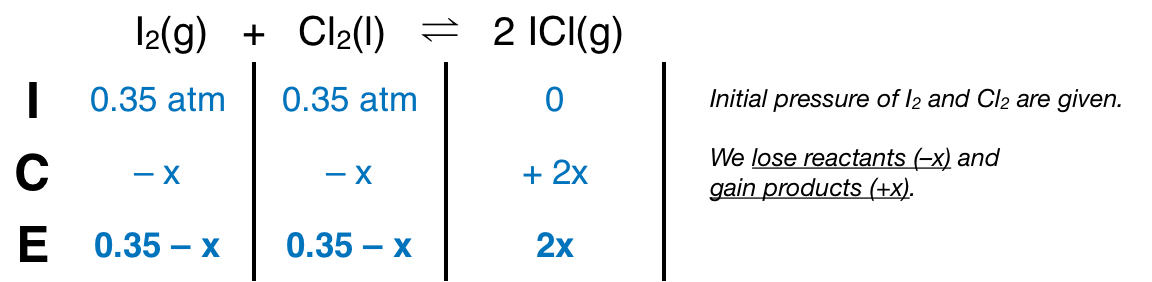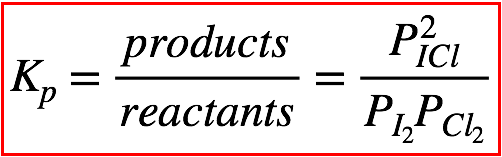# Problem: Consider the reaction between iodine gas and chlorine gas to form iodine monochloride: I2(g) + Cl2(g) ⇌ 2ICl(g); Kp = 81.9 (at 298 K)A reaction mixture at 298 K initially contains PI2 = 0.35 atm and PCl2 = 0.35 atm. What is the partial pressure of iodine monochloride when the reaction reaches equilibrium?

###### FREE Expert Solution
80% (442 ratings)
###### FREE Expert Solution

We’re given the following equilibrium reaction:

I2(g) + Cl2(g)  2 ICl(g); Kp = 81.9 (at 298 K)

We know that the initial pressure of I2 and Cl2 is 0.35 atm. From this, we can construct an ICE table:The Kp expression for the reaction is:Note that each pressure is raised by the stoichiometric coefficient: PI2 and PCl2 is raised to 1 while PICl is raised to 2. Plugging in the equilibrium pressures from the ICE table into the Kp expression:

80% (442 ratings)###### Problem Details

Consider the reaction between iodine gas and chlorine gas to form iodine monochloride:

I2(g) + Cl2(g) ⇌ 2ICl(g); Kp = 81.9 (at 298 K)

A reaction mixture at 298 K initially contains PI2 = 0.35 atm and PCl2 = 0.35 atm. What is the partial pressure of iodine monochloride when the reaction reaches equilibrium?

Frequently Asked Questions

What scientific concept do you need to know in order to solve this problem?

Our tutors have indicated that to solve this problem you will need to apply the ICE Chart concept. You can view video lessons to learn ICE Chart. Or if you need more ICE Chart practice, you can also practice ICE Chart practice problems.

What is the difficulty of this problem?

Our tutors rated the difficulty ofConsider the reaction between iodine gas and chlorine gas to...as medium difficulty.

How long does this problem take to solve?

Our expert Chemistry tutor, Dasha took 6 minutes and 36 seconds to solve this problem. You can follow their steps in the video explanation above.

What professor is this problem relevant for?

Based on our data, we think this problem is relevant for Professor Houston's class at CSUS.